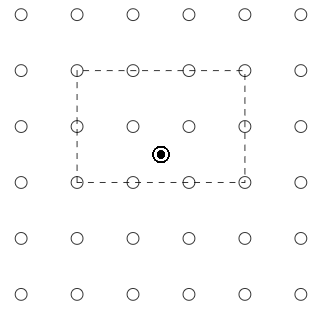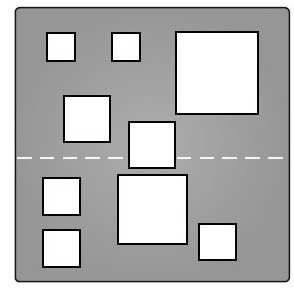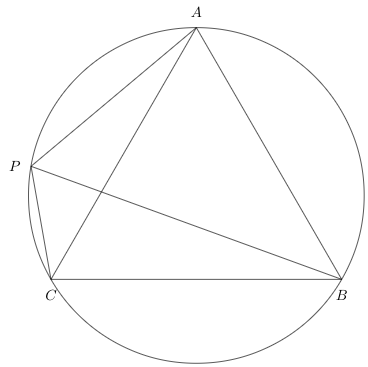MT #10: Full-syllabus

Instructions

• You are responsible for keeping time. Email all your solutions by 17:05 Hrs IST.
• Find an email from me with the subject line ‘Mock test 10: Full-syllabus’. Send your solutions (images) as replies to this email.
• Adjust/Reduce the resolution of the camera so that each image is less than 500 KB in size.
• Total marks: 100 (10x4=40 for Part A + 6x10=60 for Part B)

For students who miss the live test (members only)
Self-administer the mock test and email your solutions before 19 June, 23:59 Hrs. Your solutions will be evaluated but marks won’t be counted for official use in the future. Solutions submitted after 19 June, 23:59 Hrs will not be evaluated. As per the rules of CMI entrance exam, no calculators or log tables must be used.

Submission file: Write answers to all the ten questions on a single sheet of paper. Email a picture of your answer sheet. Name the file as PartA.jpg or PartA.png.

For this part, answers must be written without any explanation.

1. There are three pastures of area 35 acres, 10 acres and 65 acres. On a certain date the owner places 17, 7 and 23 cows in the pastures, respectively. The grass in the first pasture is exhausted after 7 weeks, and that in the second after 4 weeks. For how long can the 23 cows be grazed in the third pasture?
Assume that initially there is the same amount of grass/unit area and that new grass grows at the same constant rate in all three pastures.

2. Find the shortest distance from the point $$A=(0,2)$$ to the arc of the parabola $$y=x^2$$ which is between $$x=0$$ and $$x=1$$.

3. We have an $$n\times n$$ grid of points, where $$n$$ is an even number. There is a black spot at the center of the grid. In how many ways can we draw an axis-parallel rectangle with corners as the grid points such that the spot is inside the rectangle? An example of a rectangle is shown for a grid of size $$n=6$$:4. Let $$A$$ be a $$3 \times 3$$ matrix with entries from the set $$\{-1 , +1\}$$. Also, it is given that the first row and the first column of $$A$$ contains only $$(+1)$$. Find the maximum value of determinant of $$A$$.

5. Evaluate : $$\displaystyle\int_0^{102} \sum_{k=1}^{100} \dfrac{\prod_{n=1}^{100}(x-n)}{x-k}\,\text{dx}$$

6. Does there exist a real polynomial $$H(x)$$ with integer coefficients such that $$H(13) = 21$$ and $$H(50) = 122$$?

7. Consider two functions $$f$$ and $$g$$ defined as : $f(x) = \dfrac{1}{x+a} + \dfrac{1}{x+b} - \dfrac{1}{c_1}\quad~\&~\quad g(x) = \dfrac{1}{x+a} + \dfrac{1}{x+b} - \dfrac{1}{c_2}$ where $$a, b, c_1, c_2$$ are real constants. Both $$f$$ and $$g$$ have the property that their roots are equal in magnitude, but opposite in sign, i.e. if $$\alpha$$ is one root of $$f$$ , then its other root is $$(-\alpha)$$ . Simlarly, if $$\beta$$ is one root of $$g$$ , then the other root of $$g$$ is $$(-\beta)$$.

Let $$P_1$$ denote the product of roots of $$f$$ , and let $$P_2$$ denote the product of two roots of $$g$$. Find the value of $$\big(P_1 + P_2\big)$$ , when $$a = 12$$ , $$b = 13$$ , $$c_1 = 147$$ and $$c_2 = 187$$ .

8. Evaluate the following integral : $$\displaystyle\int_{0}^{2\pi} \frac{1}{3+\cos t}dt$$

9. We have a circular table with 6 chairs. Alice, Bob and Charlie each pick a random chair to sit down in. What is the probability that there are two empty chairs next to each person?

10. Given a complex number $$z$$ such that $$z^{13}=1$$, find all possible values of $$z+z^{3}+z^{4}+z^{9}+z^{10}+z^{12}$$.

Part B: Subjective questions

Submission files: Each question in this part must be answered on a page of its own. Name the files as B1.jpg, B2.jpg, etc. In case you have multiple files for the same question, say B4, name the corresponding files as B4-1.jpg, B4-2.jpg, etc.

Clearly explain your entire reasoning. No credit will be given without reasoning. Partial solutions may get partial credit.

B1. In how many ways can $$2^{100}$$ be expressed as a sum of four squares of integers?

B2. Consider the following polynomial where $$a_1,\ldots,a_n$$ are distinct integers. $r(x) = \left(x-a_{1}\right)^{2}\left(x-a_{2}\right)^{2}\left(x-a_{3}\right)^{2} \ldots \left(x-a_{n}\right)^{2}+1$ Prove that $$r(x)$$ cannot be written as the product of two other polynomials with integral coefficients.

B3. (i) Suppose $$f$$ is a differentiable function from the $$\mathbb{R}$$ into $$\mathbb{R}$$. Suppose $$f'(x) > f(x)$$ for all $$x \in \mathbb{R}$$ , and $$f(x_0) = 0$$ for some $$x_0 \in \mathbb{R}$$. Prove that $$f(x) > 0$$ for all $$x > x_0$$. $$\;\;\;$$ [5 marks]
(ii) Show that the equation $$Ae^x = 1 + x + \frac{x^2}{2}$$ , where $$A$$ is a positive constant, has exactly one real root. $$\;\;\;$$ [5 marks]

B4. We have a unit square made out of black paper. There are $$n$$ axis-aligned white squares contained inside the black unit square. Let $$S$$ denote the set of white squares. The squares in $$S$$ are pairwise disjoint. Prove that there exists a horizontal line that intersects the unit square and passes through at most $$\sqrt{n}$$ squares in $$S$$.
You may assume that $$n$$ is a perfect square. For example, the figure shows a unit square containing $$n=9$$ white squares.B5. $$ABC$$ is an equilateral triangle and $$P$$ is point on the circumcircle of $$ABC$$. $$P$$ is any point on the arc $$AC$$. Prove that $$PB=PA+PC$$.B6. Let $$x$$ be any real number and $$N$$ be any integer $$>1$$. Show that there exists a rational number $$p/q$$, with $$p$$ and $$q$$ integers and $$0< q \leqslant N$$, such that: $\left|x-\frac{p}{q}\right| < \frac{1}{Nq}$
For example, if $$x=\pi$$ and $$N=10$$, we can take $$p/q=22/7$$. Then $$|\pi-22 / 7|<1/70$$.